# Mathematics

### Chapter : Polynomials

#### Relation between the zero and the coefficients of a polynomials

Consider quadratic polynomial P(x) = 2x2 – 16x + 30.
Now, 2x2 – 16x + 30
= (2x – 6) (x – 3)
= 2(x – 3) (x – 5)
The zeroes of P(x) are 3 and 5.

Sum of the zeroes are = 3 + 5 = 8 =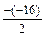Product of the zeroes are = 3 × 5 = 15 =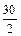So if ax2 + bx + c, a ≠ 0 is a quadratic polynomial and a, b are two zeroes of polynomial then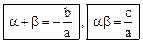Example: Find the zeroes of the quadratic polynomial 6x2 – 13x + 6 and verify the relation between the zeroes and its coefficients.

Solution: We have,
6x2 – 13x + 6
= 6x2 – 4x – 9x + 6
= 2x (3x – 2) –3 (3x – 2)
= (3x – 2) (2x – 3)
So, the value of quadratic equation 6x2 – 13x + 6 is 0, when
(3x – 2) = 0 or (2x – 3) = 0 i.e.,
When x =or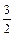Therefore, the zeroes of 6x2 – 13x + 6 areand.

Sum of the zeroes :+=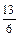=Product of the zeroes
=×=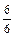=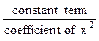Example: Find the zeroes of the quadratic polynomial 4x2 – 9 and verify the relation between the zeroes and its coefficients.

Solution: We have,
4x2 – 9
= (2x)2 – 32
= (2x – 3) (2x + 3)
So, the value ofa quadratic equation 4x2 – 9 is 0, when
2x – 3 = 0 or 2x + 3 = 0
i.e., when x =or x =.
Therefore, the zeroes of 4x2 – 9 are&.

Sum of the zeroes
=+= 0 ==Product of the zeroes
==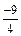Example: Find the zeroes of the quadratic polynomial 9x2 – 5 and verify the relation between the zeroes and its coefficients.

Solution: We have,
9x2 – 5
= (3x)2 – (√5)2
= (3x – √5) (3x +√5)
So, the value of 9x2 – 5 is 0, when
3x –√5 = 0 or 3x +√5 = 0
i.e., when x =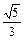or x =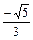.

Sum of the zeroes

== 0 ==Product of the zeroes
=×=